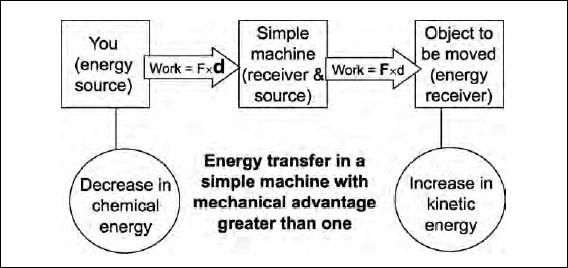# What are simple machines?

Simple machines are devices that match human capabilities to do work to tasks that need to be done. They can reduce the force required or reduce the distance or direction an object must be moved.

Suppose you want to lift a heavy object. If you use a machine you will use your stored chemical energy to do work on the machine. The machine, in turn, does work on the object, which increases its energy. If the force is constant and in the direction of motion, then work is the product of force and distance moved (W = Fd). The mechanical advantage (MA) of a simple machine is the output force divided by the input force (Foutput / Finput = MA). To make the output force larger than the input force you must choose a machine that has a mechanical advantage larger than one. The drawing below illustrates the process. Note that you exert a small input force over a large distance. The machine exerts a larger output force over a smaller distance.Because we will eventually run out of fossil fuels, we need to explore other, more sustainable forms of energy, such as solar power.
Close

This is a web preview of the "The Handy Physics Answer Book" app. Many features only work on your mobile device. If you like what you see, we hope you will consider buying. Get the App